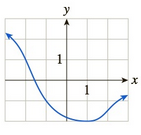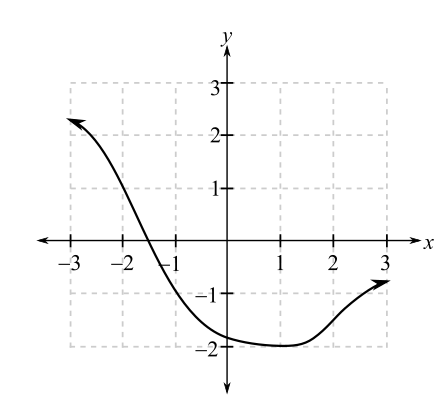Chapter 10.1, Problem 35EFinite Mathematics and Applied Cal...

7th Edition
Stefan Waner + 1 other
ISBN: 9781337274203

Solutions

Chapter
SectionFinite Mathematics and Applied Cal...

7th Edition
Stefan Waner + 1 other
ISBN: 9781337274203
Textbook Problem

In Exercises 35–48 the graph of f is given. Use the graph to compute the quantities asked for. [HINT: See Examples 4–5.]a. lim x → 1 f ( x ) b. lim x → − 1 f ( x )(a)

To determine

The value of limx1f(x) using the graph as follows:Explanation

Given information:

The graph is as follows:

From the graph, limit is determined for x tends to 1 for the function f(x).

For this, take a point on the graph to the left of x=1 and move right along the curve, so that the x-coordinate approaches 1. Then, it is noticed from the graph that the y-coordinate approaches 2.

Similarly, take a point on the graph to the right of x=1 and move along the curve to the left so that the x-coordinate approaches 1

(b)

To determine

The value of limx1f(x) using the graph which is as follows:Still sussing out bartleby?

Check out a sample textbook solution.

See a sample solution

The Solution to Your Study Problems

Bartleby provides explanations to thousands of textbook problems written by our experts, many with advanced degrees!

Get Started

Evaluate the integral, if it exists. sinxcos(cosx)dx

Single Variable Calculus: Early Transcendentals, Volume I

43. Find the slope of the line tangent to the graph of

Mathematical Applications for the Management, Life, and Social Sciences

Evaluate 6 8 24 30

Study Guide for Stewart's Multivariable Calculus, 8th

limx1x3x21x = _____. a) 1 b) 1 c) 0 d) does not exist

Study Guide for Stewart's Single Variable Calculus: Early Transcendentals, 8th

Find x so that -2,3, x,6, and 8,8 are collinear.

Elementary Geometry for College Students